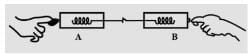Test: Newton Third Law Of Motion

# Test: Newton Third Law Of Motion - Class 9

Test Description

## 10 Questions MCQ Test Science Class 9 - Test: Newton Third Law Of Motion

Test: Newton Third Law Of Motion for Class 9 2023 is part of Science Class 9 preparation. The Test: Newton Third Law Of Motion questions and answers have been prepared according to the Class 9 exam syllabus.The Test: Newton Third Law Of Motion MCQs are made for Class 9 2023 Exam. Find important definitions, questions, notes, meanings, examples, exercises, MCQs and online tests for Test: Newton Third Law Of Motion below.
Solutions of Test: Newton Third Law Of Motion questions in English are available as part of our Science Class 9 for Class 9 & Test: Newton Third Law Of Motion solutions in Hindi for Science Class 9 course. Download more important topics, notes, lectures and mock test series for Class 9 Exam by signing up for free. Attempt Test: Newton Third Law Of Motion | 10 questions in 10 minutes | Mock test for Class 9 preparation | Free important questions MCQ to study Science Class 9 for Class 9 Exam | Download free PDF with solutions
 1 Crore+ students have signed up on EduRev. Have you?
Test: Newton Third Law Of Motion - Question 1

### A child on a cart with wheels throws a sandbag forward. As a result:

Detailed Solution for Test: Newton Third Law Of Motion - Question 1

The child moves in the backward direction as a consequence of action-reaction law. Sandbag exerts an equal and opposite force in the backward direction.

Test: Newton Third Law Of Motion - Question 2

### When a fireman directs a powerful stream of water from a hose-pipe, the hose-pipe tends to go backward. This is due to:

Detailed Solution for Test: Newton Third Law Of Motion - Question 2
• According to the circumstances mentioned in the question, a powerful stream of water on a fire from a hose-pipe tend to go backward.
• This phenomenon is the practical life example of Newton's third law, " Every action has an equal and opposite reaction."
Test: Newton Third Law Of Motion - Question 3

### Which among the following is true as per Newton’s third law:

Test: Newton Third Law Of Motion - Question 4

Action and reaction forces are always:

Detailed Solution for Test: Newton Third Law Of Motion - Question 4
• It is Newton's third law of motion which states that every action has an equal and opposite reaction.
• So, every action and reaction forces are equal and in opposite direction.
Test: Newton Third Law Of Motion - Question 5

A person standing on a weighing scale sees a reading on the scale as 148 pounds. This person is acted on by:

Detailed Solution for Test: Newton Third Law Of Motion - Question 5
• Gravitational force is acting in the downward direction. As we know every action has a reaction.
• So, there is an equal and opposite force acting in opposite direction also.
Test: Newton Third Law Of Motion - Question 6

An archer shoots an arrow. Consider the action force to be the bow string against the arrow. The reaction force is the:

Detailed Solution for Test: Newton Third Law Of Motion - Question 6

According to Newton's 3rd law of motion, " Every action has an equal and opposite reaction ".  So if we have to push the arrow in forward direction, we will pull the bow string in backward direction.

Test: Newton Third Law Of Motion - Question 7

Two spring balances pull each other in opposite directions. If the reading of one spring balance is 2 N, the reading shown by the second spring balance will be:

Detailed Solution for Test: Newton Third Law Of Motion - Question 7The spring balances are hooked and pulled in opposite direction. By the action-reaction pair force, the reading must be equal to 2.0 N.

Test: Newton Third Law Of Motion - Question 8

Which one is correct if a bullet is fired from a rifle?

Detailed Solution for Test: Newton Third Law Of Motion - Question 8
• When a bullet is fired from a rifle, the rifle exerts a force on the bullet in the forward direction. This is the action force.
• The bullet also exerts an equal force on the rifle in the backward direction. This is the reaction force.
• So because of this action-reaction pair force, the bullet moves in the forward direction and the rifle moves in the backward direction.
Test: Newton Third Law Of Motion - Question 9

Which is incorrect statement about action and reaction forces?

Detailed Solution for Test: Newton Third Law Of Motion - Question 9
• The action and reaction force always act on different objects.
• Two forces acting on the same object, even if they have the same magnitude and point in the opposite direction, never form an action-reaction pair.
Test: Newton Third Law Of Motion - Question 10

A player is catching a ball. Consider the action force to be the impact of the ball against the player’s glove. What is the reaction force?

Detailed Solution for Test: Newton Third Law Of Motion - Question 10

Reaction force: Force that the glove exerts on the ball is the reaction force, opposite to the impact of the ball on the player’s glove.

## Science Class 9

66 videos|352 docs|97 tests
Information about Test: Newton Third Law Of Motion Page
In this test you can find the Exam questions for Test: Newton Third Law Of Motion solved & explained in the simplest way possible. Besides giving Questions and answers for Test: Newton Third Law Of Motion, EduRev gives you an ample number of Online tests for practice

## Science Class 9

66 videos|352 docs|97 tests